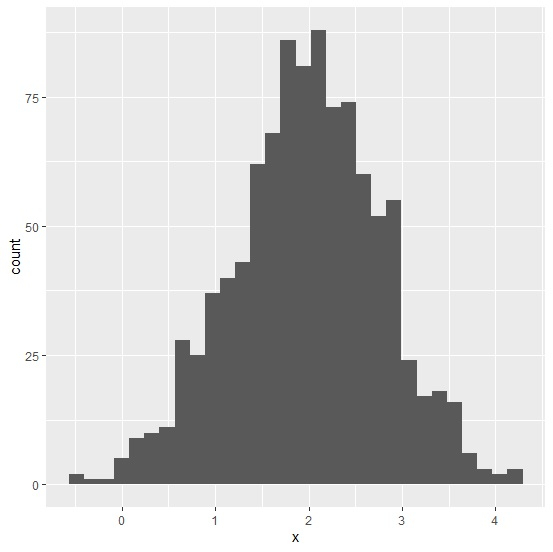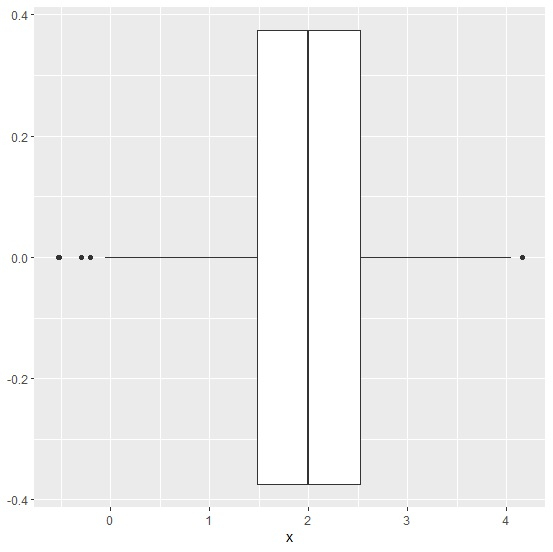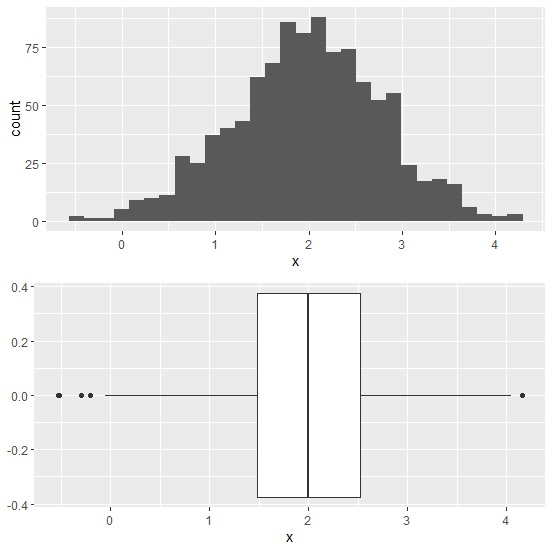# How to create two plots using ggplot2 arranged in a vertical manner in R?

R ProgrammingServer Side ProgrammingProgramming

The two plots created by using ggplot2 can be arranged in a vertical manner with the help gridExtra package, we simply needs to use grid.arrange function to do so. For example, if we have two plots created by using ggplot2 and saved in objects p1 and p2 then they can be vertically arranged as grid.arrange(p1,p2)

Consider the below data frame −

## Example

set.seed(151)
x<-rnorm(1000,2,0.80)
df<-data.frame(x)

library(ggplot2) ggplot(df,aes(x))+geom_histogram(bins=30)

## Outputggplot(df,aes(x))+geom_boxplot()

## Outputlibrary(gridExtra)
grid.arrange(p1,p2)